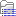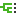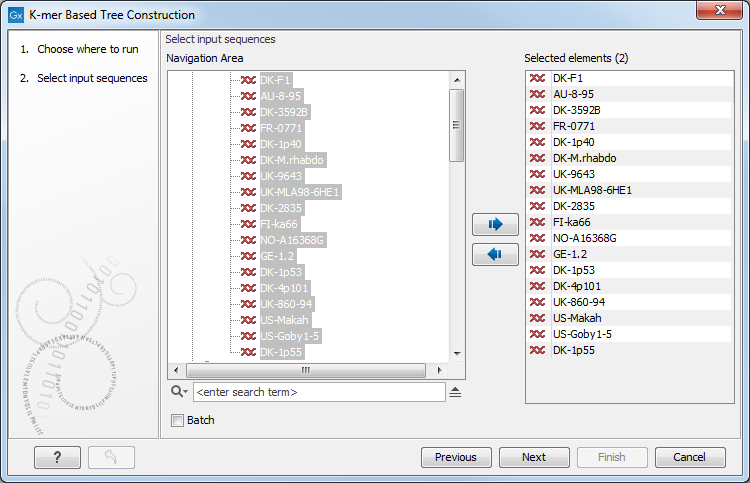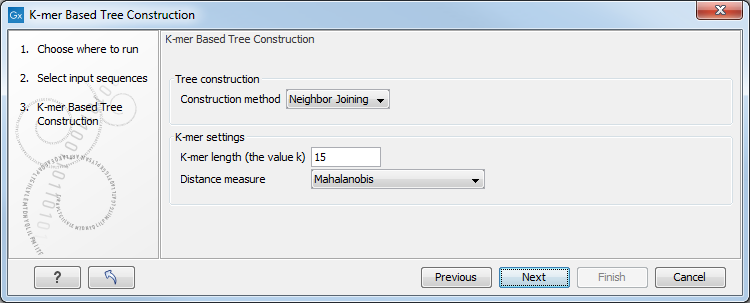# K-mer Based Tree Construction

The "K-mer Based Tree Construction" uses single sequences or sequence lists as input and is the simplest way of creating a distance-based phylogenetic tree. To run the "K-mer Based Tree Construction" tool:

Toolbox | Classical Sequence Analysis () | Alignments and Trees ()| K-mer Based Tree Construction ()

Select sequences or a sequence list (figure 20.1):Figure 20.1: Select sequences needed for creating a tree with K-mer based tree construction.

Next, select the construction method, specify the k-mer length and select a distance measure for tree construction (figure 20.2):Figure 20.2: Select the construction method, and specify the k-mer length and a distance measure.

• Tree construction
• Tree construction method The user is asked to specify which distance-based method to use for tree construction. There are two options (see Distance-based methods):
• The UPGMA method. Assumes constant rate of evolution.
• The Neighbor Joining method. Well suited for trees with varying rates of evolution.

• K-mer settings
• K-mer length (the value k) Allows specification of the k-mer length, which can be a number between 3 and 50.
• Distance measure The distance measure is used to compute the distances between two counts of k-mers. Three options exist: Euclidian squared, Mahalanobis, and Fractional common K-mer count. See 20.4.1 for further details.